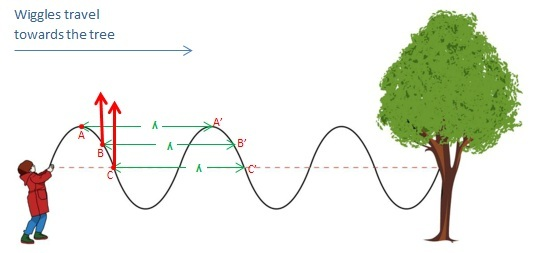# Relationship Between Wavelength and Frequency

## Wavelength and Frequency – Relationship

Waves can be described using a number of different characteristics of a wave. Wavelength and frequency are two such characteristics. The relationship between wavelength and frequency is that the frequency of a wave multiplied by its wavelength gives the speed of the wave, as we will see below.

## What is Wavelength

We have discussed what wavelength means when we were discussing the difference between Wavelength and Period. To recap; every point on a wave is oscillating. That is, every point on a wave shows some kind of regular, repetitive change in some value. For example, if you create a wave by wiggling a rope up and down, then the molecules that make up the rope are repeatedly moving up and down. If you take an electromagnetic wave, the value of electric and magnetic fields due to the wave at a point is always changing. If the wave in question is not just a short pulse, then at any given time, there can be several points on a wave that are at the same stage of oscillation. For instance, two points on the wave that reach their maximum value in oscillation simultaneously are oscillating in unison. Such points, which are always at the same stage in oscillation are said to be in phase with each other.  Wavelength is the distance between the two closest points that are in phase with each other along a wave. Thus, two adjacent peaks or two adjacent troughs on a wave are separated by a distance of one wavelength. Often, we use the Greek letter lambda ($\lambda$) to represent the wavelength of a wave:Wavelength of a wave created by wiggling a rope up and down

Note that I have referred to wavelength as the shortest distance: this is a mere technicality because there are infinitely many paths that one could take to move from one point to another. Some definitions of wavelength may not specifically mention the shortest path but, in this case, the shortest distance is implied in the definition.

## What is Frequency

Frequency ($f$) is the number of complete oscillations that a wave undergoes per unit time. It is measured in units of hertz (Hz). For sound waves, the frequency is related to the pitch of the sound. The higher the frequency, the higher is the pitch. For instance, the “middle C” note is a sound wave with a frequency of 261.63 Hz. This means that to produce this note, molecules creating or transmitting the sound wave should oscillate 261.63 times every second. The note middle D which has a higher pitch than the middle C has a frequency of 293.66 Hz. Humans can hear sounds with frequencies between about 20 – 20000 Hz. Sounds with frequencies lower than the audible range is called infrasound and the sounds with frequencies above the human hearing range are called ultrasound.

## What is the relationship between Wavelength and Frequency

You could create a wave with a higher frequency on a rope by wiggling up and down at a faster rate. As you do this, you will notice that the wavelength of the wave becomes shorter. Clearly, there is a relationship between wavelength and frequency and now we will try to find out exactly what this relationship is.

Period ($T$is another quantity that we can use to characterize a wave. Period is the time taken for one complete oscillation. Since frequency measures the number of times that a wave oscillates per unit time, it follows that$f=\frac{1}{T}$

Since a wave undergoes one complete oscillation during one period, all points in the wave return back to the same values after one period. This happens as a result of each “stage of oscillation” traveling a distance of one wavelength during one period to end up at a point that was at the same stage of oscillation one period earlier. In other words, during one period, a crest travels to the position that its preceding crest was occupying one period before, and so on.

The speed of the wave ($v$) is the distance that the wave travels per unit time. Considering that the wave travels a distance of one wavelength during one period,$v=\frac{\lambda}{T}$

We know that$T=\frac{1}{f}$. So we can write the above equation as:$v=f\lambda$

That is, the speed of a wave is equal to its frequency multiplied by the wavelength. This is the relationship between wavelength and frequency.

Electromagnetic waves traveling through vacuum have a speed of 3×108 m s-1. This speed is a fundamental constant in physics, and it is denoted by the letter$c$. So, this equation is sometimes written as$c=f\lambda$ for electromagnetic travelling through vacuum.

This equation is very useful. For example, we know that electromagnetic waves can slow down when they travel from air into glass. The frequency of the wave is determined by the original disturbance that caused the wave, so the frequency does not change when the wave goes from one medium to another. Since$v=f\lambda$, this means that in order to  maintain the same frequency while the speed is decreasing, the wavelength of the wave must be reduced as well.The speed and wavelength of a wave changes when it travels from one medium into another.

This is explained with an animation in the video below:

Image Courtesy

“Wave in a rope.” by CK-12 Foundation (File:High School Chemistry.pdf, page 178) [CC BY-SA 3.0], via Wikimedia Commons (Modified)